# [PYTHON] Julia Quick Note  Complex Numbers

### Complex number (example of writing)

#### `note03`

``````
The imaginary unit is represented by im.
z = 4 + 3im

◆ Real part
real(z)

◆ Imaginary part
imag(z)

◆ Complex conjugate
conj(z)

◆ Absolute value
abs(z)
abs2(z)… A function that gets the square of the absolute value

``````

## Commentary

In mathematics, the complex number \$ z \$ is generally expressed as follows, with the complex number unit being \$ i \$, \$ x \$ and \$ y \$ as real numbers.

``````z = x + iy
``````

In Julia, the imaginary unit can be \$ im \$ as well to represent complex numbers. For example, if the real part is 3 and the imaginary part is 4, it will be as follows.

``````z = 4 + 3im
``````

◆ You can get the real part (real part) with real (z).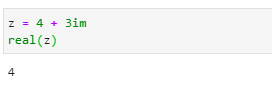◆ You can get the imaginary part (imaginary part) with imag (z).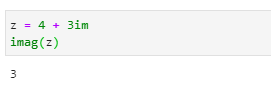◆ To get the complex conjugate, use conj (z).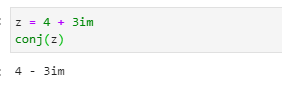◆ Absolute value of complex number|z|To get abs(z)Use the.There is also a function ** abs2 () ** to get the square of the absolute value. This is convenient if you want to take the square.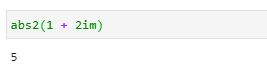: point_up_2: Julia allows you to perform four arithmetic operations on complex numbers.

addition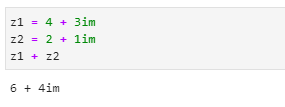subtraction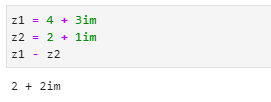multiplication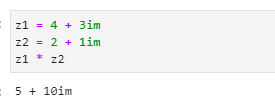division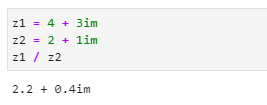◆ Confirm by numerical calculation that ① below holds.

``````z = 1 +As 2im\\
zz^* = x^2 + y^2 = |z|^2　　... ①
``````

Calculating \$ zz ^ * \$ gives:

``````zz^* = (1 + 2im) * conj(1 + 2im) = 5
``````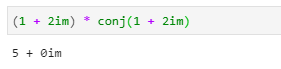Also, if you calculate \$ x ^ 2 + y ^ 2 \$, it will be as follows.

``````x^2 + y^2 = 1 + 2^2 = 5
``````

Finally,|z|^2Is calculated as follows.Therefore, ① was confirmed.

## Lottery

Julia Quick Look Note  How to use variables and constants Julia Quick Look Note  Arithmetic Expressions, Operators [Julia Quick Note  Complex Numbers] (https://qiita.com/ttabata/items/225c77a4d71fafc3e482) Julia Quick Look Note  Regular Expression [Julia quick note  if statement] (https://qiita.com/ttabata/items/4f0bcff1e32f60402dfb)

(* We will continue to increase the content)

## Related information

: paperclip: Julia --Official page https://julialang.org/

: paperclip: Julia --Japanese official document https://julia-doc-ja.readthedocs.io/ja/latest/index.html

: paperclip: First time Julia and installation (Windows & Linux) https://qiita.com/ttlabo/items/b05bb43d06239f968035

:paperclip: Julia - Mathematics https://docs.julialang.org/en/v1/base/math/

## Opinions etc.

If you have any opinions or corrections, please let us know.